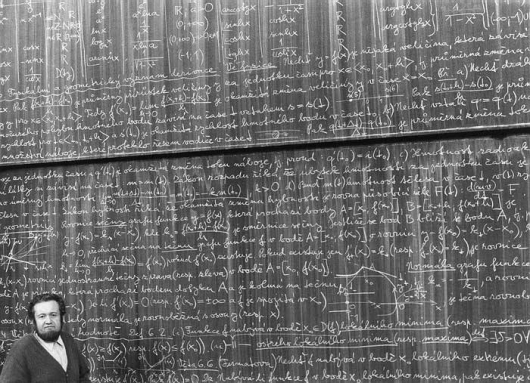Posted in Science & Nature

# Quod Erat Demonstrandum

1. Let a and b be equal non-zero quantities
a = b

2. Multiply by a
a = ab²

3. Subtract b²
a² – b² = ab – b²

4. Factor both sides
(a – b)(a + b) = b(a – b)

5. Divide out (a – b)
a + b = b

6. Observing that a = b
b + b = b

7. Combine like terms on the left
2b = b

8. Divide by b
2 = 1

9. Add 1 to each side and flip over equation

1 + 1 = 3

Q.E.D. (Thus we have proved)

How is this proof possible?The source of the fallacy is the fifth step, where (a – b) is divided out.

As a = b, a – b = 0, and dividing by zero is impossible in mathematics.